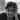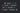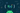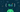Navigate back to the homepageHari Vignesh Jayapalan
June 29th, 2020 · 2 min readThis post showcases couple of creative ways to compose a function. It leverages the power of extension functions and operator overloading

### Function composition

Function composition is a technique to compose a function using multiple functions. This will allow us to achieve the following.

• Keep the functions follow single responsibility principle
• Prevents mutation within the function
• Making the function easily testable
• Makes the program safer.

If you would like to learn more about function composition or functional programming, you can refer the following series

Functional Prgramming in Kotlin

Let’s take a look on how a function composition is done on a usual way. For the sake of simplicity, I’m going to consider a simple square and triple example.

`.css-1chxjt6{position:absolute;right:22px;top:24px;padding:8px 12px 7px;border-radius:5px;color:#6f7177;-webkit-transition:background 0.3s ease;transition:background 0.3s ease;}.css-1chxjt6:hover{background:rgba(255,255,255,0.07);}.css-1chxjt6[data-a11y="true"]:focus::after{content:"";position:absolute;left:-2%;top:-2%;width:104%;height:104%;border:2px solid var(--theme-ui-colors-accent,#6166DC);border-radius:5px;background:rgba(255,255,255,0.01);}@media (max-width:45.9375em){.css-1chxjt6{display:none;}}1fun square(value: Int): Int = value * value2fun triple(value: Int): Int = 3 * value34fun compose(5    square: (Int) -> Int,6    triple: (Int) -> Int7): (Int) -> Int = { value -> square(triple(value)) }89val squareOfTriple: (Int) -> Int = compose(::square, ::triple)1011println(squareOfTripleOp(3)) // output -> 81`

This above composition is all well and good. But you may want to replace the property function `squareOfTriple` with an actual function. One such scenario is when we need to overload the function. Such as,

`1object Math {23    fun calculate(value: Int): Int = square(triple(value))45    // we obviously don't need a new function just for this Long type6    // consider this example only for understading the situation7    fun calculate(value: Long): Int = square(triple(value.toInt()))89    private fun square(value: Int): Int = value * value10    private fun triple(value: Int): Int = 3 * value11}`

For such situations, we cannot use functional references, as our function `calcualte()` is no longer a property function + we cannot overload the property based on return value. So, we need to use functions for sure.

if we want to use reusable composition with functional references, we can avoid the use of `square(triple(value))` like the example below

`1object Math {23    fun calculate(value: Int): Int = 4        compose(::square, ::triple).invoke(value)56    fun calculate(value: Long): Int = 7        compose(::square, ::triple).invoke(value.toInt())89    private fun square(value: Int): Int = value * value10    private fun triple(value: Int): Int = 3 * value1112    private fun compose(13        square: (Int) -> Int,14        triple: (Int) -> Int15    ): (Int) -> Int = { value -> square(triple(value)) }16}`

### Creative compositon using extensions

The function composition mentioned in the previous section is perfectly fine. But let’s get a bit more creative. To make you understand the final result, I’m going to go ahead and break the spoilers. Our final outcome should be like the following

`1object Math {2    3    fun calculate(value: Int): Int = 4        ::square.then(::triple)(value)56    fun calculate(value: Long): Int = 7        ::square.then(::triple)(value.toInt())89    // we can chain as much as we can ->10    // ::square.then(::triple).then(::square)1112}`

Interesting right? Let’s get to work.

To achieve this, we need to create an extension function for the function of type ’(?) -> ?’ of name ‘then’ which composes our square of triple for us

`1fun <T, U, V> ((T) -> U).then(other: (U) -> V): (T) -> V = 2    { other(this(it)) }`

with the above extension function `then()` we can get the following compostion `::square.then(::triple)(value)`

But why stop here? Let’s take our creativity a one step further. As before, let’s set our expectation

`1object Math {2    3    fun calculate(value: Int): Int = 4        (::square then ::triple)(value)56    fun calculate(value: Long): Int = 7        (::square then ::triple)(value.toInt())89}`

If you have wrestled with extension functions in kotlin, you know where I’m getting at. Yes, you are right! the `infix` function

`1infix fun <T, U, V> ((T) -> U).then(other: (U) -> V): (T) -> V = 2    { other(this(it)) }`

Kotlin allows us to provide implementations for a predefined set of operators on our types. These operators have fixed symbolic representation (like + or *) and fixed precedence. To implement an operator, we provide a member function or an extension function with a fixed name, for the corresponding type, i.e. left-hand side type for binary operations and argument type for unary ones. Functions that overload operators need to be marked with the operator modifier. (source)

Now that we know what overloading an operator means, let’s get creative and put that in motion. Asusal, here’s our expectation

`1object Math {2    3    fun calculate(value: Int): Int = 4        (::square + ::triple)(value)56    fun calculate(value: Long): Int = 7        (::square + ::triple)(value.toInt())89}`

To achieve this, we need to create an extension function for the function of type ’(?) -> ?’ by overloading the operator plus ’+’ which composes our square of triple for us

`1operator fun <T, U, V> ((T) -> U).plus(other: (U) -> V): (T) -> V = 2    { other(this(it)) }`

By overloading the `plus` operator, we were able to achieve our expectation. Feel free to overload your favorite operator like `rangeTo` etc.

### Final thougts

Personally, as much as overloading an operator is fun for this usecase, this might confuse people a lot and it might send wrong signal for overloading the operator. I’m comfortable to use extension function. Rest, I’ll leave the decision to you!

I hope you found this piece like an art to admire! If you think you have learned something new or if you like this series or you want to boost my morale, please share the post.

### More articles from Hari Vignesh Jayapalan### Functional Programming in Kotlin - f(4)

Currying Function

May 23rd, 2020 · 2 min read### Functional Programming in Kotlin - f(3)

Function Composition

May 14th, 2020 · 2 min read